## Products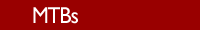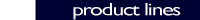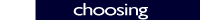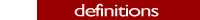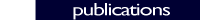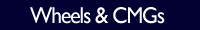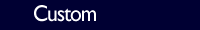### Ambient Temperature Range

The ambient temperature range is the temperature of the environment within which the MTBs are designed to operate. The MTBs are assumed to be in a vacuum and mounted to a thermal heat sink at the ambient temperature and assumed to be free to radiate to a radiative heat sink (typically the spacecraft) at the ambient temperature. The MTBs are designed to operate over the ambient temperature range of -34 to +71°C. Other temperature ranges can be accommodated. The MTBs produce the guaranteed moment while remaining below the de-rated thermal limit within the ambient temperature and bus voltage ranges.

### Bus Voltage

The nominal bus voltage required to power each MTB is specified in the suffix of its name. Thus, the MTB-002S-05V is designed to be used with a 5 V bus, while the MTB-800S-28 V is designed to be used with a 28 V bus. In general, the smaller MTBs have a bus voltage range of 5V +/- 5%, while the larger MTBs have a bus voltage range of 28 V +/- 6 V. Other bus voltages can be accommodated. The moment is smallest at the minimum bus voltage and maximum ambient temperature. The coil temperature is guaranteed to be below its de-rated thermal limit at maximum bus voltage and maximum ambient temperature. top of page

### De-rated Thermal Limit

The maximum allowed internal temperature of the MTB is in accordance with the 30 C de-rating requirements of TOR-2008(8583)-8215 and Mil-Std-1547. This temperature is limited by the potting material, rated to 130°C (the magnet wire is rated to 200°C). The de-rated thermal limit for the MTB is 100°C. This limits the power that can be applied at the maximum ambient temperature of 71°C. The maximum internal temperature of the MTBs is designed to remain below 100°C at 71°C and maximum bus voltage applied continuously. top of page

### Hysteresis

Hysteresis is the variation in the moment dependent upon the history of the current applied to it. The magnetic moment at a given current will be greater if the current is decreasing than if it is increasing. This effect generates the residual moment. top of page

### Magnetic Dipole Moment

The current applied to the windings magnetizes the core, generating a magnetic moment measured in Ampere-meter squared (Am2). This moment interacts with the Earth’s magnetic field to generate a torque applied to the spacecraft, controlling its attitude. The torque generated is given by:

Torque = Moment x B-Field

Where Torque, Moment and B-field are all vectors. See also moments. top of page

### Moments

Design Moment: the moment that the MTB was designed to produce. Each MTB is named for its design moment.

• The design moment is guaranteed at minimum bus voltage and 25°C.

• Ninety percent (90%) of the design moment is guaranteed at minimum bus and 71°C.

• Non-Linearity is typically 10% or less at the design moment.

Guaranteed Moment: the moment the MTB is guaranteed to produce in vacuum between -34 and +71°C over the defined bus voltage range. The moment is smallest at minimum bus voltage and maximum ambient temperature.

Linear Moment: the moment below which the non-linearity is nominally 5% of itself. The non-linearity is guaranteed to be less than 5% over 90% of the linear range.

Linear Range: – Linear Moment to + Linear Moment

Saturation Moment: the moment at which the non-linearity is nominally30% of the linear moment. The non-linearity is guaranteed to be less than 30% over 90% of the saturation range. The saturation moment defines the moment the MTB can be expected to produce if enough current is applied.

Saturation Range: – Saturation Moment to + Saturation Moment

Residual Moment: the magnetic moment produced by the MTB when no power is applied due to magnetic hysteresis in the core material. The residual moment will have the polarity last applied to the MTB in the absence of an external magnetic field. The Earth’s magnetic field along the length of the MTB tends to magnetize the MTB. This effect can dominate the residual moment. top of page

### Non-Linearity

For moments less than the linear moment, the magnetic moment is approximately the product of the scale factor and the current. The difference between the actual moment and this product is the non-linearity, traditionally expressed as a percentage of the linear moment. At the linear moment, the non-linearity is approximately 5%. As the current increases, the magnetic moment will continue to increase, but the curve will start to flatten out as the moment increases up to the saturation moment and beyond. At the saturation moment, the non-linearity is approximately 30%. top of page

### Scale Factor

The scale factor is determined by a least square fit of the data within a range where the non-linearity is expected to be less than 1% (typically +/- half of the design moment). When the magnetic moment is less than the linear moment, the moment increases approximately linearly with the current. The scale factor is the slope of the moment versus current data for small currents (i.e. the slope of the tangent line). top of page